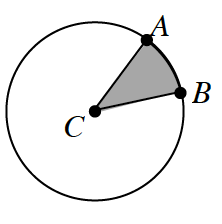### Home > GC > Chapter 10 > Lesson 10.1.4 > Problem10-40

10-40.The spinner at right is designed so that if you randomly spin the spinner and land in the shaded sector, you win $\1{,}000{,}000$. Unfortunately, if you land in the unshaded sector, you win nothing. Assume point $C$ is the center of the spinner.

1. If $m∠ACB=90°$, how many times would you have to spin to reasonably expect to land in the shaded sector? How did you get your answer?

$4 \; \text{times}$
2. What if $m∠ACB=1°$? How many times would you have to spin to reasonably expect to land in the shaded sector?
3. Suppose $P \left(\text{winning} \; \1{,}000{,}000\right) = \frac{1}{5}$ for each spin. What must $m∠ACB$ equal? Show how you got your answer.
$\frac{360°}{5}=72°$